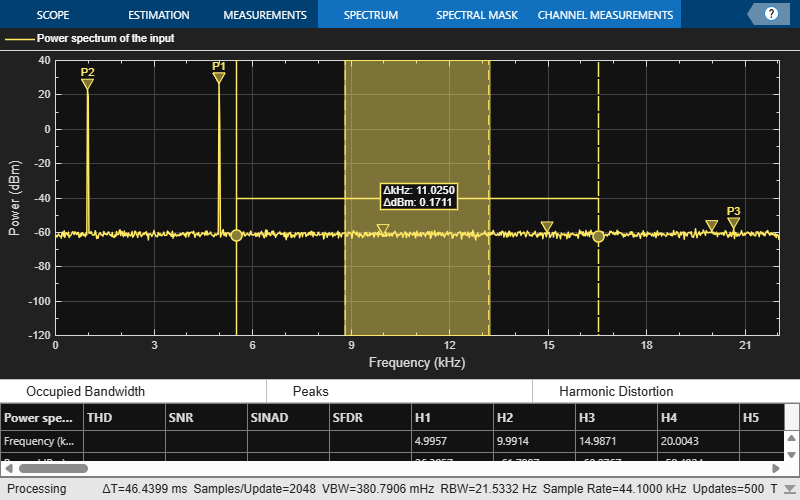# Obtain Measurement Data Programmatically for `dsp.SpectrumAnalyzer` System object

Compute and display the power spectrum of a noisy sinusoidal input signal using the `dsp.SpectrumAnalyzer` System object. Measure the peaks, cursor placements, adjacent channel power ratio, distortion, and CCDF values in the spectrum by enabling the following properties:

• `PeakFinder`

• `CursorMeasurements`

• `ChannelMeasurements`

• `DistortionMeasurements`

• `CCDFMeasurements`

### Initialization

The input sine wave has two frequencies: 1000 Hz and 5000 Hz. Create two `dsp.SineWave` System objects to generate these two frequencies. Create a `dsp.SpectrumAnalyzer` System object to compute and display the power spectrum.

```Fs = 44100; Sineobject1 = dsp.SineWave('SamplesPerFrame',1024,'PhaseOffset',10,... 'SampleRate',Fs,'Frequency',1000); Sineobject2 = dsp.SineWave('SamplesPerFrame',1024,... 'SampleRate',Fs,'Frequency',5000); SA = dsp.SpectrumAnalyzer('SampleRate',Fs,'Method','Filter bank',... 'SpectrumType','Power','PlotAsTwoSidedSpectrum',false,... 'ChannelNames',{'Power spectrum of the input'},'YLimits',[-120 40],'ShowLegend',true);```

### Enable Measurements Data

To obtain the measurements, set the `Enable` property of the measurements to `true`.

```SA.CursorMeasurements.Enable = true; SA.ChannelMeasurements.Enable = true; SA.PeakFinder.Enable = true; SA.DistortionMeasurements.Enable = true;```

### Use `getMeasurementsData`

Stream in the noisy sine wave input signal and estimate the power spectrum of the signal using the spectrum analyzer. Measure the characteristics of the spectrum. Use the `getMeasurementsData` function to obtain these measurements programmatically. The `isNewDataReady` function indicates when there is new spectrum data. The measured data is stored in the variable `data`.

```data = []; for Iter = 1:1000 Sinewave1 = Sineobject1(); Sinewave2 = Sineobject2(); Input = Sinewave1 + Sinewave2; NoisyInput = Input + 0.001*randn(1024,1); SA(NoisyInput); if SA.isNewDataReady data = [data;getMeasurementsData(SA)]; end end```The right side of the spectrum analyzer shows the enabled measurement panes. The values shown in these panes match with the values shown in the last time step of the `data` variable. You can access the individual fields of `data` to obtain the various measurements programmatically.

### Compare Peak Values

Peak values are obtained by the `PeakFinder` property. Verify that the peak values obtained in the last time step of `data` match the values shown on the spectrum analyzer plot.

`peakvalues = data.PeakFinder(end).Value `
```peakvalues = 3×1 26.9850 24.1735 -52.3506 ```
`frequencieskHz = data.PeakFinder(end).Frequency/1000`
```frequencieskHz = 3×1 4.9957 0.9905 7.8166 ```